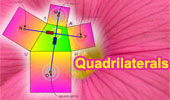The Incas used trapezoids for all their windows and doors, which withstand earthquakes well.

# Quadrilaterals: Theorems and Problems 6Proposed Problem 170. Trapezoid, Midpoint, Triangle, Area.

Proposed Problem 169. Parallelogram, Interior and Exterior Points, Diagonals, Pentagon, Triangles, Areas

Proposed Problem 168. Parallelogram, Diagonal, Triangles, Pentagon, Areas.

Proposed Problem 167. Parallelogram, Diagonal, Triangles, Areas.

Proposed Problem 166. Parallelogram, Diagonal, Triangles, Areas.

Proposed Problem 165. Parallelogram, Diagonal, Triangles, Areas.

Proposed Problem 164. Parallelogram, Trapezoid, Diagonal, Triangles, Areas.

Proposed Problem 163. Trapezoid, Diagonals, Triangles, Areas.

Proposed Problem 162. Parallelogram, Triangles, Areas.

Proposed Problem 161. Parallelogram, Midpoints, Octagon, Areas.

Proposed Problem 153. Circumscribed Quadrilateral, Diagonals Concurrent with Chords.

Proposed Problem 152. Circumscribed Quadrilateral, Diagonal, Chord, Proportion.

Proposed Problem 151. Quadrilateral, Area, Trisection of Sides.

Proposed Problem 150. Quadrilateral, Area, Trisection of Sides.

Proposed Problem 149. Quadrilateral, Area, Midpoints.

Proposed Problem 148. Quadrilateral, Area, Midpoints.

Proposed Problem 147. Quadrilateral, Area, Midpoints, Triangle.

Proposed Problem 146. Varignon's Theorem: Quadrilateral, Midpoints, Parallelogram, Area, Perimeter.

Proposed Problem 139. Triangle Area, Orthic Triangle, Semiperimeter, Circumradius.

Proposed Problem 137. Orthic Triangle, Altitudes, Perpendicular, Incircle, Collinear Points, Parallelogram.

Trapezoid, Triangle, Diagonals, Midpoints. Dynamic Geometry.
Step-by-Step construction, Manipulation, and animation.
Prove proposition.

Four Circles Theorem Using Interactive Dynamic Software
Step-by-Step construction, Manipulation, and animation. Cyclic Quadrilateral.

The Pythagorean Curiosity Triangles and squares, fifteen conclusions

Inca Skull Surgery
Inca Skull Surgeons Were "Highly Skilled," Study Finds.
Trepanation = square hole in the skull.

Triangle and Squares Theorem 1: with Interactive Geometry Software
Step-by-Step construction, Manipulation, and animation.

Bottema's Theorem: Triangle and Squares with Interactive Geometry Software
Step-by-Step construction, Manipulation, and animation.

Eight-Point Circle Theorem
Step-by-Step construction, Manipulation, and animation.

Using TracenPoche Dynamic Software
Step-by-Step construction, Manipulation, and animation

Parallelogram Problem 96. Similar Triangles, Incenters, Parallelogram.

Parallelogram Problem 93. Similar Triangles, Circumcircles, Parallelogram.

Proposed Problem 90. Quadrilateral and Triangle Areas, Midpoints.

Proposed Problem 88. Triangle and Quadrilateral Areas, Midpoints.

Proposed Problem 87. Area of Quadrilaterals and Midpoints of Diagonals.

Newton's Theorem, Newton-Gauss Line: Complete quadrilateral theorem. Using TracenPoche Dynamic Geometry Software, Online Step-by-Step construction, manipulation, and animation.

Proposed Problem 78: Angles of a Circle. Perpendicular and parallel lines, Midpoint, Diameter, Chord, Cyclic quadrilateral, Congruence.

Proposed Problem 77: Angles of a Circle. Parallel lines, Cyclic quadrilateral.

Square
Proposed Problem 76: Area of a Circle
. Square, Circle, Circular Sector.

Proposed Problem 74: Three Intersecting Circles. Cyclic quadrilateral, Angles.

Proposed Problem 73: Three Intersecting Circles. Cyclic quadrilateral.

Proposed Problem 72: Intersecting Circles. Cyclic quadrilateral, Chords, Parallel.

Four Circles Theorem Using TracenPoche Dynamic Software
Step-by-Step construction, Manipulation, and animation.

Proposed Problem 63: Heptagon Regular, Side and Diagonals.

Proposed Problem 62: Square Diagonal, Inscribed Circle.

Arbelos, Inscribed Circle and Concyclic Points Cyclic Quadrilateral.

Proposed Problem 57: Angle bisector, circles Cyclic Quadrilateral.

Proposed Problem 55: Angle bisector, circles Cyclic Quadrilateral.

Eyeball Theorem: Animated Angle to Geometry Study.

Varignon and Wittenbauer parallelograms. Quadrilateral: midpoints and trisection points of the edges.

Van Aubel's Theorem. Quadrilateral with Squares. Proof with animation.

Brahmagupta's Formula  Area of a cyclic quadrilateral.

Brahmagupta Formula Extension Area of any quadrilateral.

Newton's Theorem: Newton's Line. Circumscribed quadrilateral, midpoints of diagonals, center of the circle inscribed.

Puzzle of the Newton's Theorem: 50 pieces of circles.

Sangaku Problem. The incenters of four triangles in a cyclic quadrilateral form a rectangle.

Kurschak's Tile and Theorem. Jozsef Kurschak (Hungary, 1864-1933) An elegant and a purely geometric way of finding the area of a regular dodecagon.

Proposed Problem 33.